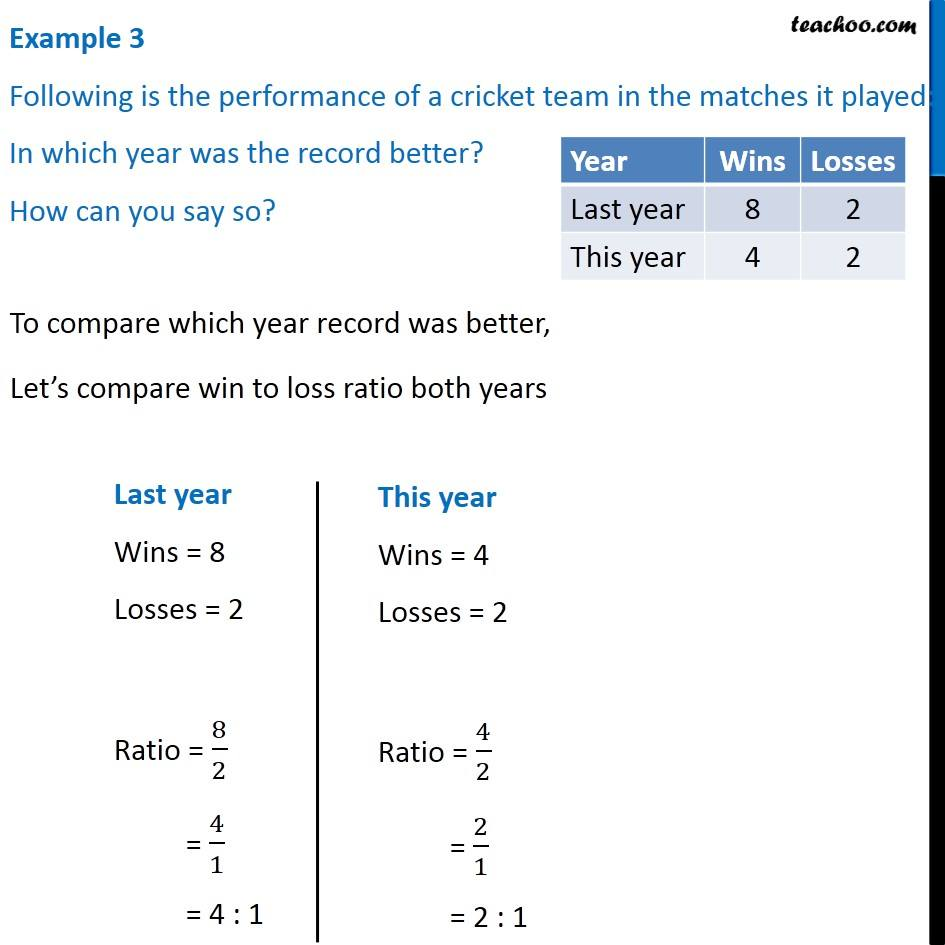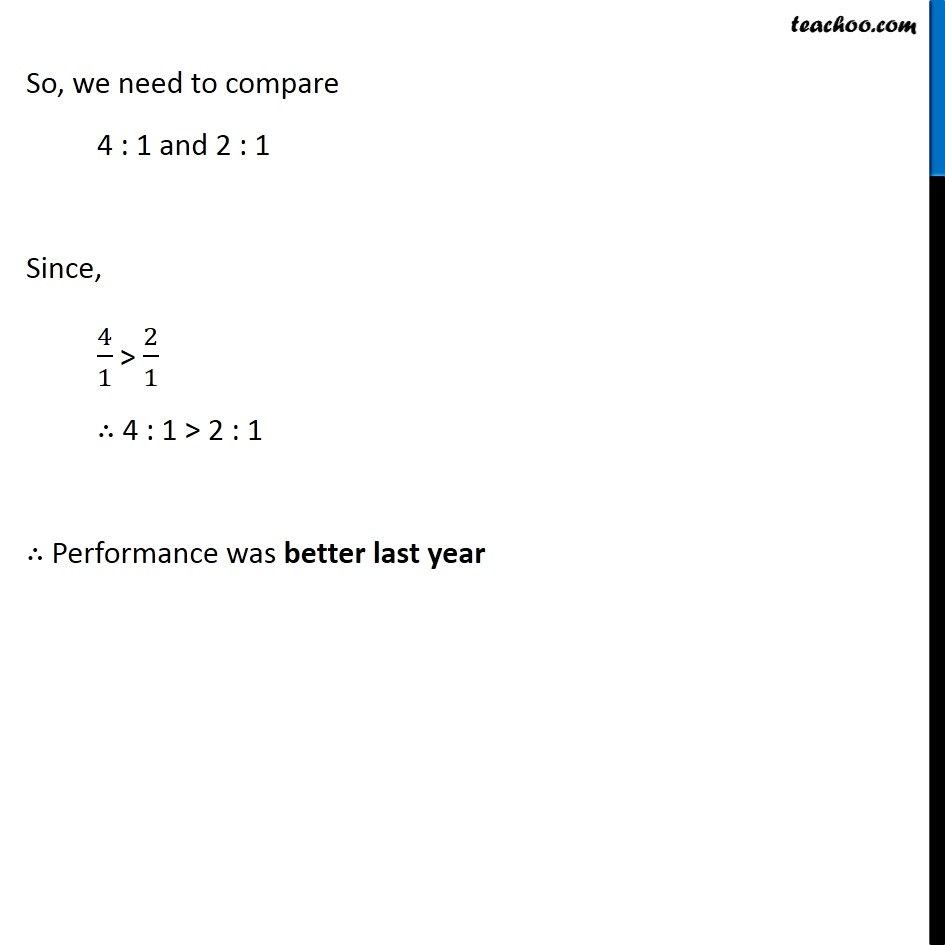Examples

Chapter 8 Class 7 Comparing Quantities
Serial order wise### Transcript

Example 3 Following is the performance of a cricket team in the matches it played: In which year was the record better? How can you say so? To compare which year record was better, Let’s compare win to loss ratio both years Last year Wins = 8 Losses = 2 Ratio = 8/2 = 4/1 = 4 : 1 This year Wins = 4 Losses = 2 Ratio = 4/2 = 2/1 = 2 : 1 So, we need to compare 4 : 1 and 2 : 1 Since, 4/1 > 2/1 ∴ 4 : 1 > 2 : 1 ∴ Performance was better last year